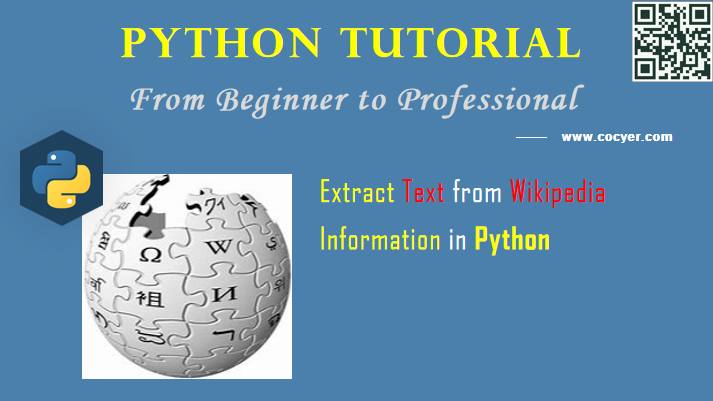# Python: Extract Text from Wikipedia

Wikipedia is an important text resources for nlp. In this tutorial, we will introduce how to extract text from it using python.1.Install wikipedia library

`pip install wikipedia`

2.Import library

`import wikipedia`

3.Get article summary

`print(wikipedia.summary("Python Programming Language"))`

We also can limit the length of summary by sentence.

`wikipedia.summary("Python programming languag", sentences=2)`

Run this code, you will print 2 sententces.

4.Search terms

```result = wikipedia.search("Neural networks")
print(result)```

Run this code, you will get these search results:

`['Neural network', 'Artificial neural network', 'Convolutional neural network', 'Recurrent neural network', 'Rectifier (neural networks)', 'Feedforward neural network', 'Neural circuit', 'Quantum neural network', 'Dropout (neural networks)', 'Types of artificial neural networks']`

```page = wikipedia.page('Neural network')
# get the title of the page
title = page.title
# get the categories of the page
categories = page.categories
content = page.content
# get all the links in the page